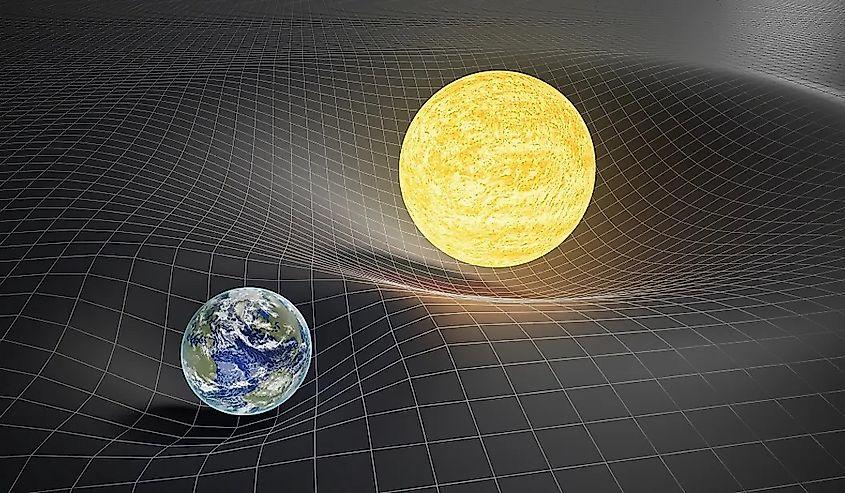# The Four Forces Of Physics

The universe is held together by the laws of physics. All the laws of physics are dictated by four forces: gravity, electromagnetism, the weak nuclear force, and the strong nuclear force. These four forces hold our universe together, and without them, the universe could not exist. Each of the four forces, with the exception of gravity, have their own messenger particles that carry the force. What are these forces and how do they operate?

## GravityVisual representation of how gravity is the warping of space

Gravity is perhaps the most well-known of the four forces. Not only was it the earliest force to be identified, but it also operates on the largest scales, making it far more apparent than the other three forces. Gravity was first described by Isaac Newton in the 1660s. Newton sought to describe how objects fall to the Earth, and why other objects, such as the moon, orbit the Earth. Newton devised a mathematical model that described how objects with mass exert a force of attraction, a force he called gravity. The force of gravity is proportional to the mass of an object, with higher mass objects extorting a stronger gravitational force. Interestingly, despite the success of Newton’s theory, he was unable to define what gravity actually was. He knew it was a force of attraction, yet he did not know why objects exerted a gravitational force. It wouldn’t be until the early 20th century, when Albert Einstein published his theory of gravity called General Relativity, that we would have a definition for how gravity is produced. Einstein was able to describe how the gravitational force works. Rather than being a mysterious force between objects, gravity is the warping of space itself. Space can be thought of as a fabric, wherein objects of mass can distort and bend the fabric. For example, the sun creates a kind of indent in space, and all the planets orbit along that curved space. Interestingly, despite gravity being the most recognizable of the four forces, it is by far the weakest force in the universe.

Although Einstein was able to describe what gravity is, it still remains the most mysterious of the four forces. With the advent of quantum mechanics in the early 20th century, the world of physics was thrown into chaos. Most of what we thought was true turned out to be wrong, and the universe proved to be far more mysterious than we could have ever imagined. In this new world of quantum mechanics, gravity has had a hard time finding its place. The other three forces of nature can be described using quantum mechanics, yet no matter how hard scientists try, gravity cannot be accurately described in the quantum realm.

## ElectromagnetismElectromagnetism is carried by light, which forms the electromagnetic spectrum.

After gravity, electromagnetism is perhaps the most popular force in the universe. All of us likely experience it everyday. By reading this on a phone, computer, or tablet, you are using the electromagnetic force. Our entire global civilization is powered by electromagnetism. Interestingly, electromagnetism was once considered to be two separate forces: electricity and magnetism. The first scientist to identify a connection between these two forces was Micheal Faraday. In the early 1800s, Micheal Faraday realized that an electric current could induce a magnetic field, and that a magnetic field could induce an electric current. He correctly identified that, rather than being two separate forces, electricity and magnetism were actually manifestations of the same force, which he called electromagnetism. Unfortunately, Faraday lacked the mathematical knowledge to describe electromagnetism, and so his theory was not widely accepted. Then, in 1865, another scientist by the name of James Clerk Maxwell built upon Faraday’s ideas. Maxwell went on to describe the electromagnetic force in terms of mathematics, creating the famous Maxwell equations. In addition to combining electricity and magnetism under one roof, Maxwell found that light is the messenger of the electromagnetic force.

## The Strong And Weak Nuclear Forces

The weak nuclear force is a relatively recent discovery. Its existence was first proposed by physicist Enrico Fermi, who proposed the existence of the weak force in 1933. The weak force describes the interaction of atoms in terms of radioactive decay, and without it, things like nuclear fission and nuclear fusion could not occur. Although it is called the weak force, it is still significantly stronger than gravity. We do not notice the weak force in our everyday lives due to the fact that it operates on extremely tiny scales. Although the existence of the weak force was first proposed in 1933, its messenger particles, called W and Z bosons, were not confirmed until the 1980s. Furthermore, in the 1960s, scientists were able to combine both the electromagnetic force and the weak force into a single force called the electroweak force.

The strong nuclear force is the most recent force to be discovered. Up until the 1970s, scientists could not describe how atoms themselves are held together. To explain how atoms stay together rather than fly apart, scientists proposed the existence of the strong nuclear force. The strong force is carried by a subatomic particle called a gluon. As their name suggests, they “glue” atoms together. The strong nuclear force is the strongest of the four forces, despite the fact it operates on the smallest scales.

Share